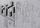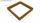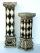Prism + unit conversion - examples

1. Triangular prismCalculate a triangular prism if it has a rectangular triangle base with a = 4cm and hypotenuse c = 50mm and height of the prism is 0.12 dm.
2. Prism 4 sidesThe prism has a square base with a side length of 3 cm. The diagonal of the sidewall of the prism/BG/is 5 cm. Calculate the surface of this prism in cm square and the volume in liters
3. PrismRight angle prism, whose base is right triangle with leg a = 7 cm and hypotenuse c = 10 cm has same volume as a cube with an edge length of 1 dm. a) Determine the height of the prism b) Calculate the surface of the prism c) What percentage of the cube
4. PoolMr. Peter build a pool shape of a four-sided prism with rhombus base in the garden. Base edge length is 8 m, distance of the opposite walls of the pool is 7 m. Estimated depth is 144 cm. How many hectoliters of water consume Mr. Peter to fill the pool?
5. TetrapackHow high should be the milk box in the shape of a prism with base dimensions 8 cm and 8.8 cm if its volume is 1 liter?
6. Milk packageMilk is sold in a box with dimensions of 9.5 cm; 16.5 cm and 6.5 cm. Determine the maximum amount of milk that can fit into a box. Coating thickness is negligible.
7. Pine woodFrom a trunk of pine 6m long and 35 cm in diameter with a carved beam with a cross-section in the shape of a square so that the square had the greatest content area. Calculate the length of the sides of a square. Calculate the volume in cubic meters of lum
8. Paper boxCalculate the consumption of paper on the box-shaped quadrangular prism with rhombic footstall, base edge a=6 cm and the adjacent base edges forms an angle alpha = 60 °. Box height is 10 cm. How many m2 of the paper consumed 100 such boxes?
9. Building baseExcavation for the building base is 350x600x26000. Calculate its volume in m3.
10. The tankThe tank has 1320 liters of water. The tank has the shape of a prism, its base is an rectangle with sides a = 0,6 m and b = 1,5 m. How high does the water level reach in the tank?
11. PrismThe volume of tetrahedral prism is 2.43 m3. Base of prism is a parallelogram in which a side 2,5dm and height ha = 18cm. Calculate the height of the prism.
12. CanisterGasoline is stored in a cuboid canister having dimensions 44.5 cm, 30 cm, 16 cm. What is the total weight of a full canister when one cubic meter of gasoline weighs 710 kg and the weight of empty canister is 1.5 kg?
13. Tetrahedral prism - rhomboid baseCalculate the area and volume tetrahedral prism that has base rhomboid shape and its dimensions are: a = 12 cm, b = 70 mm, v_a = 6 cm, v_h = 1 dm.
14. TentCalculate how many liters of air will fit in the tent that has a shield in the shape of an isosceles right triangle with legs r = 3 m long the height = 1.5 m and a side length d = 5 m.
15. FlowerbedThe flowerbed has a length 3500mm and a width 1400mm. How many foil is needed to covers the flowerbed? How many m2 of foil was consumed for its production (add 10% of the material to the joint and waste)? How many liters of air is inside the enclosure? (F
16. Surface and volume od cuboidContent area of the square base of cuboid is Sp = 36 cm2 and its height 80 mm. Determine its surface area and volume.
17. Regular triangular prismCalculate the surface area of body of regular triangular prism, when the length of its base edge is 6.5 cm and height 0.2 m.
18. Support columCalculate the volume and surface of the support column that is shaped as perpendicular quadrangular prism whose base is a rhombus with a diagonals u1 = 102 cm u2 = 64 cm. Column height is 1. 5m.
19. PrismCalculate the height of the prism having a surface area 448.88 dm² wherein the base is square with a side of 6.2 dm. What will be its volume in hectoliters?
20. Surface areaCalculate the surface area of a four-sides 2-m high prism which base is a rectangle with sides 17 cm and 1.3 dm

Do you have an interesting mathematical example that you can't solve it? Enter it, and we can try to solve it.

To this e-mail address, we will reply solution; solved examples are also published here. Please enter e-mail correctly and check whether you don't have a full mailbox.